Latest Banking jobs   »

# Quantitative Aptitude Quiz For SBI PO Mains 2023- 20th January

Q1. Quantity I: Height of the tank if the volume of cylindrical tank is 12320 cubic cm. Its radius and height are in the ratio of 7 : 10 respectively.
Quantity II: Level kerosene in the jar. A conical vessel of base radius 2 cm and height 3 cm is filled with kerosene. This liquid leaks through a hole in the bottom and collects in a cylindrical jar of radius 2 cm.

(a) Quantity I > Quantity II
(b) Quantity I < Quantity II
(c) Quantity I ≥ Quantity II
(d) Quantity I ≤ Quantity II
(e) Quantity I = Quantity II or No relation

Q2. A man who swim 48m/minute in still water, swims 200m against the current and 200m with the current. The difference between the time taken by him against the stream and with the stream is 10 minutes.
Quantity I: speed of current.
Quantity II: Speed of a man who completes 3 rounds of a circular path of radius 49 m in 14 minutes.

(a) Quantity I > Quantity II
(b) Quantity I < Quantity II
(c) Quantity I ≥ Quantity II
(d) Quantity I ≤ Quantity II
(e) Quantity I = Quantity II or No relation

Q3. Quantity I: Marked price (in rs.) of an article if a tradesman gives 4% discount on the marked price and 1 article free with every 15 articles bought and still gains 35%. C.P. of the article is Rs. 100.
Quantity II: Profit (in rs.) made on selling the pen at marked price. Even after reducing the marked price of a pen by Rs. 32, a shopkeeper makes a profit of 15%. The cost price of a pen is Rs. 320.
(a) Quantity I > Quantity II
(b) Quantity I < Quantity II
(c) Quantity I ≥ Quantity II
(d) Quantity I ≤ Quantity II
(e) Quantity I = Quantity II or No relation

Q4. Quantity I – How many three digits positive integers, with digits x, y and z exist such that x < y and z < y (Assume that ‘x’ is in hundred’s place, ‘y’ is in ten’s place and ‘z’ is in unit’s place).
Quantity II – Set A comprises all multiples of four less than 500, set B comprises all odd multiples of seven less than 500 and Set C comprises all multiples of six less than 500. How many elements are present in (A ∪ B ∪ C)
(a) Quantity I ≥ Quantity II
(b) Quantity I < Quantity II
(c) Quantity I > Quantity II
(d) Quantity I ≤ Quantity II
(e) Quantity I = Quantity II or No relation

Directions (5-8): The following questions are accompanied by two statements I and II. You have to determine which statements(s) is/are sufficient/necessary to answer the questions.

Q5. Find the sum of present age of X & Z?
I. Four years ago, average age of X, Y and Z was 16 years while age of X, Y and Z is in arithmetic progression.
II. Average of present age of X and Z is same as present age of Y while Y is four years younger than Z.
(a) Statement I alone is sufficient to answer the question but statement II alone is not sufficient to answer the question.
(b) Statement II alone is sufficient to answer the question but statement I alone is not sufficient to answer the question.
(c) Both the statements taken together are necessary to answer the questions, but neither of the statements alone is sufficient to answer the question.
(d) Either statement I or statement II by itself is sufficient to answer the question.
(e) Statements I and II taken together are not sufficient to answer the question.

Q6. Is Rahul meet Prabhat at half of the distance on a circular track (48km) if they both started to move at same time in same direction?
I. Speed of Prabhat is 24km/hr more than speed of Rahul.
II. Prabhat’s speed is 200% more than that of Rahul
(a) Statement I alone is sufficient to answer the question but statement II alone is not sufficient to answer the question.
(b) Either statement I or statement II by itself is sufficient to answer the question.
(c) Both the statements taken together are necessary to answer the questions, but neither of the statements alone is sufficient to answer the question.
(d) Statement II alone is sufficient to answer the question but statement I alone is not sufficient to answer the question.
(e) Statements I and II taken together are not sufficient to answer the question.

Q7. Find number of yellow balls in a box which contains 3 black balls and 7 red and yellow balls.
I. Probability of selecting two yellow balls from the box is 2/15
II. Probability of selecting one red and black ball is 1/5.
(a) Statement I alone is sufficient to answer the question but statement II alone is not sufficient to answer the question.
(b) Statement II alone is sufficient to answer the question but statement I alone is not sufficient to answer the question.
(c) Both the statements taken together are necessary to answer the questions, but neither of the statements alone is sufficient to answer the question.
(d) Either statement I or statement II by itself is sufficient to answer the question.
(e) Statements I and II taken together are not sufficient to answer the question.

Q8. Find number of pens sold by retailer if he earns total 37.5% profit on selling some pens and some pencils.
I. Ratio between pen sold to pencil sold is 2 : 3 while ratio between cost price of pen to pencil is 3 : 2.
II. On selling a pen and a pencil, he earns 25% and 50% profit respectively.
(a) Both the statements taken together are necessary to answer the questions, but neither of the statements alone is sufficient to answer the question.
(b) Statements I and II taken together are not sufficient to answer the question.
(c) Statement II alone is sufficient to answer the question but statement I alone is not sufficient to answer the question.
(d) Statement I alone is sufficient to answer the question but statement II alone is not sufficient to answer the question.
(e) Either statement I or statement II by itself is sufficient to answer the question.

Directions (9-10): The following questions are accompanied by two statements (I) and (II). You have to determine which statement(s) is/are sufficient / necessary to answer the questions.
Q9. Ratio between radius and height of a right circular cylinder is 3 : 2. Find area of a square?
I . Given that side of square is equal to the radius of cylinder.
II . Height of cylinder is equal to the radius of circle whose area is 616 cm2.
(a) Only statement I is sufficient
(b) Only statement II is sufficient
(c) Statement I and II both
(d) Either statement I or Statement II alone sufficient
(e) Neither statement I nor statement II sufficient

Q10. Ratio between length of two trains is 7 : 6. What will be difference between lengths of both trains?
I . Speed of smaller train is 108 km/hr and it can cross an unmoving man in 2.7 sec.
II . Time taken by larger train to cross the smaller train is 5.4 sec and speed of larger train is 90 km/hr.
(a) Only statement I is sufficient
(b) Only statement II is sufficient
(c) Statement I and II both
(d) Either statement I or Statement II alone sufficient
(e) Neither statement I nor statement II sufficient

Solutions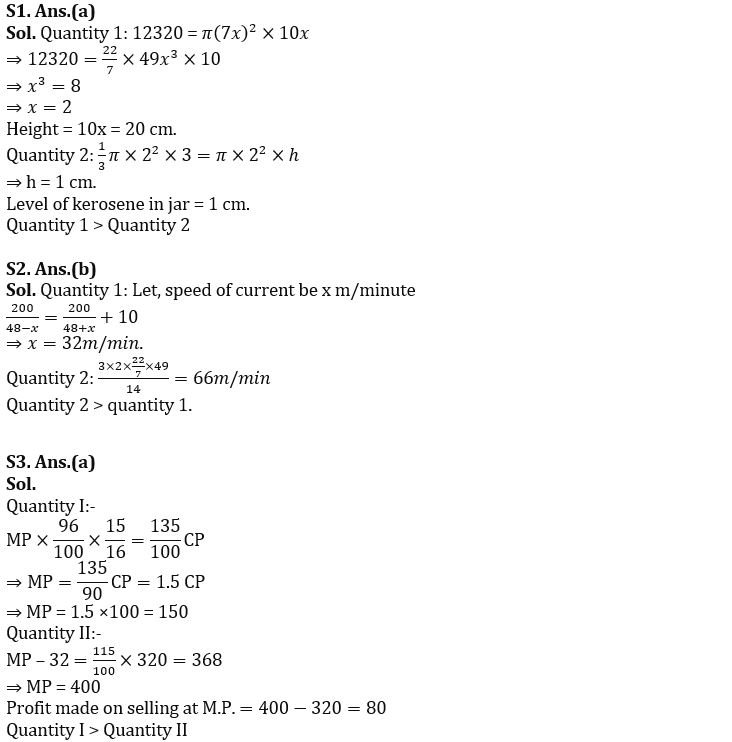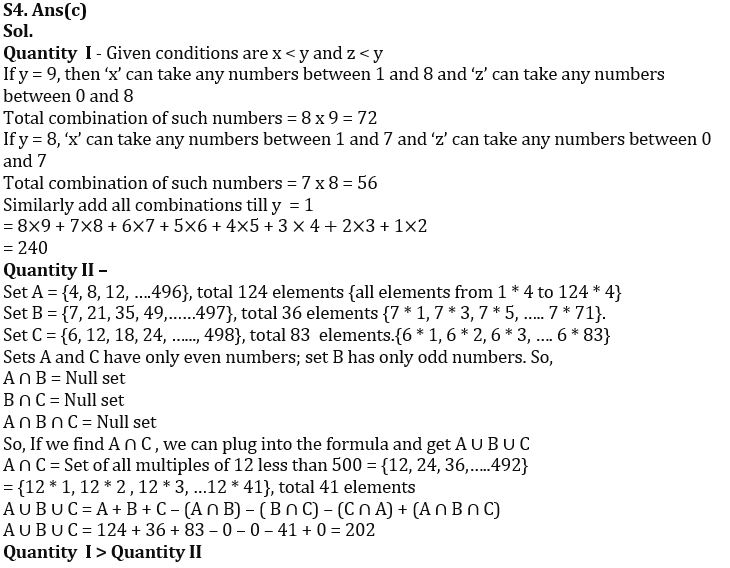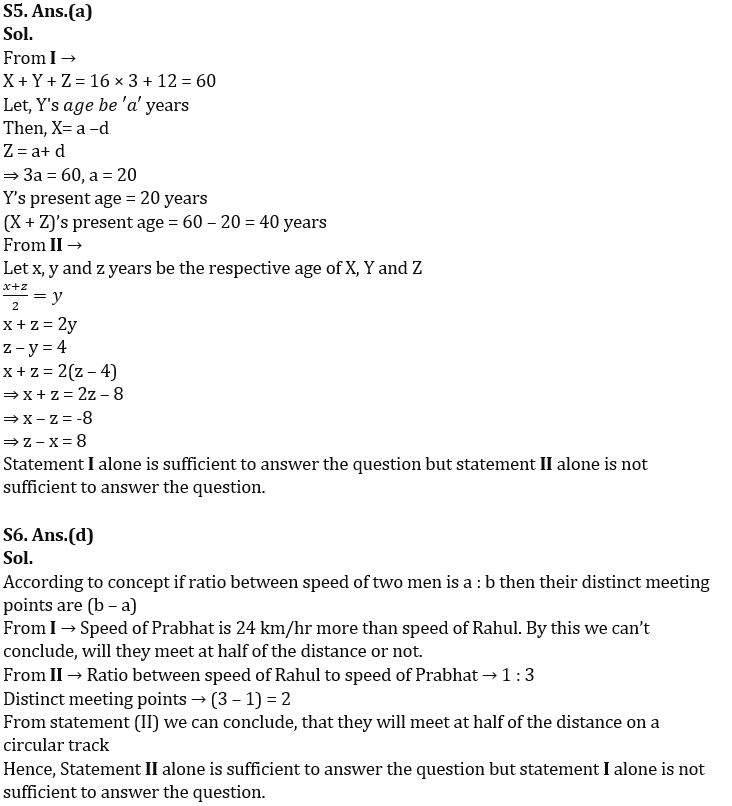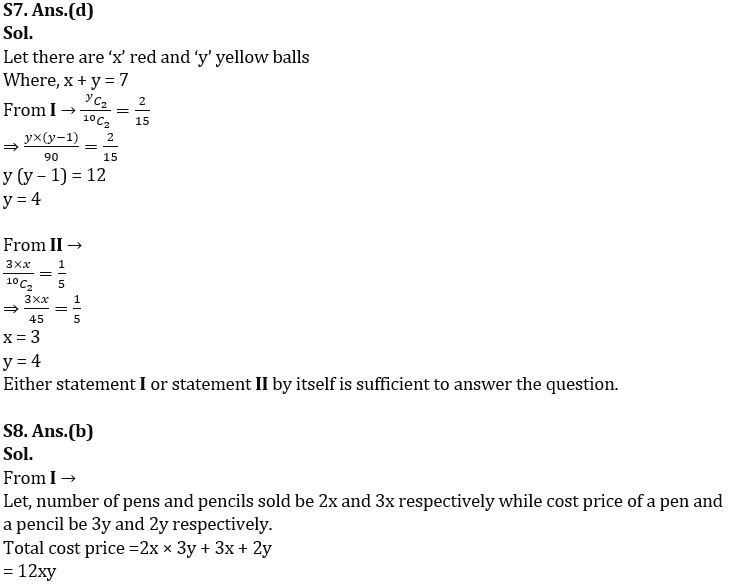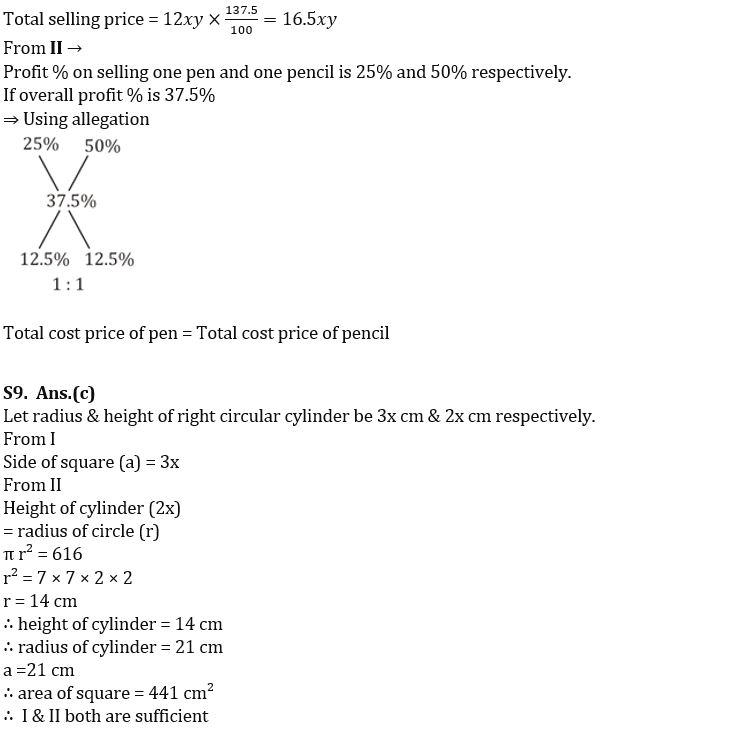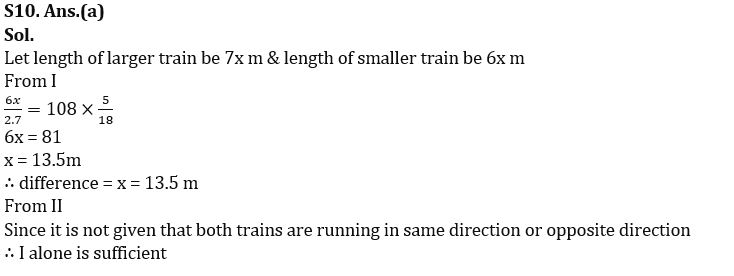## FAQs

### When is the SBI Clerk and PO Mains Exam 2022-23?

SBI Clerk & PO Mains exam are scheduled to be held on 15th & 30th January 2023.

#### Congratulations!